﻿ 具有异质主体的市场分数模型分析及实证研究 Market Fraction Model with Heterogeneous Agents and Its Empirical Study

Statistics and Application
Vol.06 No.04(2017), Article ID:22507,12 pages
10.12677/SA.2017.64052

Market Fraction Model with Heterogeneous Agents and Its Empirical Study

Lin Li*, Ke Shi#

College of Mathematics and System Science, Xinjiang University, Urumqi Xinjiang

*通讯作者。

#第一作者。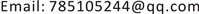Received: Oct. 8th, 2017; accepted: Oct. 24th, 2017; published: Oct. 30th, 2017ABSTRACT

On the basis of Xuezhong He and Youwei Li (2015), in this paper, we empirically evaluate the market fraction asset pricing model with heterogeneous traders. By conducting econometric analysis via Monte Carlo Simulations, we show that the estimates of the power law decay indies, GARCH parameters, TGARCH parameters, and explain the long range dependence volatility clustering, asymmetry in high-frequeny financial time series respectively. This paper did empirical research on the shenzhen composite, and the model results are compared with the results of the real market, the result show that the heterogeneous beliefs model can well describe the real market.

Keywords:Heterogeneous Agents, Monte-Carlo Simulations, Power-Lawer Decay, GARCH, TGARCH1. 引言

Brock and Hommes (1997, 1998)   提出异质代理模型的关键成分是一类非理性正反馈交易者，也称为图表分析者。图表分析者认为过去价格的变化会一直持续，和基本面者面者形成对比，基本面者基于均值回归策略，认为市场价格会不断回归基础价值，而图表分析者基于技术分析，认为价格会延续历史价格模式。经典框架下基本面者和理性交易者很相似，相比之下图表分析者就像势头交易者。除采用基本面者——图表分析者的区别外，HAMs的特征还有交易者基于过去表现改变策略的灵活性，就如Brock and Hommes (1997)  介绍的那样。在这种机制的支持下，Bloomfield and Hales (2002)  给出了一些实证证据，交易者基于最新实现利润的条件预测不断改变，即使他们明白价格基本过程是服从随机游走的。在过去20年里，有关HAMs的文献明显增多，Zeeman (1974)  首次提出用HAMs解释金融市场动力系统，这篇文献很快被Day and Huang (1990)  ，Kirman (1991)  ，Chiarella (1992)  ，Lux and Marchesi (1999)  进行推广，Brock and Hommes (1997, 1998)介绍了交易者策略转换的概念，而且在金融市场行为的解释方法引发了广泛的兴趣，参见Chiarella et al. (2009)  对相关文献的总回顾中。

He and Li (2007)  发现异质代理、学习过程中的趋势追随调整速度、噪声和基本确定动态的相互作用是幂律分布波动的原因，He and Li (2015)  验证了DAX30指数的幂率行为，并基于Monte-carlo模拟估计模型的幂率衰减指数，(FI) GARCH参数，说明模型可以很好地反映真实市场。

2. 模型的建立

2.1. 做市商机制下的市场分数和市场出清价格

${p}_{t}$ 为t时点风险资产的每股价格(不包括红利)， $\left\{{D}_{t}\right\}$ 为随机分红过程，则t + 1时h类投资者财富为

${W}_{h,t+1}=R{W}_{h,t}+\left[{p}_{t+1}+{D}_{t+1}-R{P}_{t}\right]{z}_{h,t},$ (2.1)

${R}_{t+1}={P}_{t+1}+{D}_{t+1}-R{P}_{t}.$ (2.2)

${E}_{h,t}\left({W}_{t+1}\right)=R{W}_{t}+{E}_{h,t}\left({R}_{t+1}\right){z}_{h,t},\text{\hspace{0.17em}}\text{\hspace{0.17em}}{V}_{h,t}\left({W}_{t+1}\right)={z}_{h,t}{}^{2}{V}_{h,t}\left({R}_{t+1}\right).$ (2.3)

${z}_{h,t}=\frac{{E}_{h,t}\left({R}_{t+1}\right)}{{a}_{h}{V}_{h,t}\left({R}_{t+1}\right)}.$ (2.4)

${z}_{e,t}\equiv {n}_{1}{z}_{1,t}+{n}_{2}{z}_{2,t}=\frac{1+m}{2}\frac{{E}_{1,t}\left[{R}_{t+1}\right]}{{a}_{1}{V}_{1,t}\left[{R}_{t+1}\right]}+\frac{1-m}{2}\frac{{E}_{2,t}\left[{R}_{t+1}\right]}{{a}_{2}{V}_{2,t}\left[{R}_{t+1}\right]}.$ (2.5)

${P}_{t+1}={P}_{t}+\mu {z}_{e,t}+\stackrel{˜}{{\delta }_{t}}.$

${P}_{t+1}={P}_{t}+\frac{\mu }{2}\left[\left(1+m\right)\frac{{E}_{1,t}\left[{R}_{t+1}\right]}{{a}_{1}{V}_{1,t}\left[{R}_{t+1}\right]}+\left(1-m\right)\frac{{E}_{2,t}\left[{R}_{t+1}\right]}{{a}_{2}{V}_{2,t}\left[{R}_{t+1}\right]}\right]+\stackrel{˜}{{\delta }_{t}}.$ (2.6)

2.2. 基本面者

${P}_{t+1}^{\ast }={P}_{t}^{\ast }\left[1+{\sigma }_{\epsilon }\stackrel{˜}{{\epsilon }_{t}}\right],\text{\hspace{0.17em}}\text{\hspace{0.17em}}\stackrel{˜}{{\epsilon }_{t}}\sim N\left(0,1\right),\text{\hspace{0.17em}}{\sigma }_{\epsilon }\ge 0,\text{\hspace{0.17em}}{P}_{0}^{\ast }=\overline{P}>0.$ (2.7)

$\stackrel{˜}{{\epsilon }_{t}}$ 与噪声需求 $\stackrel{˜}{{\delta }_{t}}$ 相互独立，这种模式设定确保厚尾和波动聚集现象不是由基础价值过程产生的。因此，在后面的讨论中，任何风险资产自相关结构的出现将是由交易过程自身产生的。

${E}_{1,t}\left({P}_{t+1}\right)={P}_{t}+\alpha \left({P}_{t+1}^{\ast }-{P}_{t}\right),\text{\hspace{0.17em}}\text{\hspace{0.17em}}{V}_{1,t}\left({P}_{t+1}\right)={\sigma }_{1}^{2}.$ (2.8)

2.3. 趋势追随者

${E}_{2,t}\left({P}_{t+1}\right)={P}_{t}+\gamma \left({P}_{t}-{u}_{t}\right),\text{\hspace{0.17em}}\text{\hspace{0.17em}}{V}_{2,t}\left({P}_{t+1}\right)={\sigma }_{1}^{2}+{b}_{2}{V}_{t}.$ (2.9)

${u}_{t}=\delta {u}_{t-1}+\left(1-\delta \right){p}_{t},$ (2.10)

${v}_{t}=\delta {v}_{t-1}+\delta \left(1-\delta \right){\left({P}_{t}-{u}_{t-1}\right)}^{2},$ (2.11)

$\delta \in \left[0,1\right]$ 是常数，当记忆滞后长度趋于无穷大时，样本期望和方差是个几何衰减受限过程。从根本上说，几何衰减概率过程 $\left(1-\delta \right)\left\{1,\delta ,{\delta }^{2},\cdots \right\}$ 与历史价格 $\left\{{P}_{t},{P}_{t-1},{P}_{t-2},\cdots \right\}$ 是相关联的。参数 $\delta$ 是几何衰减率。选择这个过程有两点原因：第一，估计样本均值和方差时，交易者倾向于对当前价格附以很大权重，对以前或者更早的价格附以很小的权重；第二，我们相信几何衰减过程可能有确定的自相关结构，甚至是在真实金融市场观察到的长记忆特征，另外，它也有数学处理的优势。

2.4. 完整的随机模型

${E}_{1,t}\left({R}_{t+1}\right)={P}_{t}+\alpha \left({P}_{t+1}^{\ast }-{P}_{t}\right)+\overline{D}-R{P}_{t}=\alpha \left({P}_{t+1}^{\ast }-{P}_{t}\right)-\left(R-1\right)\left({P}_{t}-\overline{P}\right),$

${V}_{1,t}\left({R}_{t+1}\right)=\left(1+q\right){\sigma }_{1}^{2}.$

${z}_{1,t}=\frac{1}{{a}_{1}\left(1+q\right){\sigma }_{1}^{2}}\left[\alpha \left({P}_{t+1}^{\ast }-{P}_{t}\right)-\left(R-1\right)\left({P}_{t}-\overline{P}\right)\right].$ (2.12)

${z}_{1,t}=\frac{\left(\alpha +R-1\right)\left(\overline{P}-{P}_{t}\right)}{{a}_{1}\left(1+q\right){\sigma }_{1}^{2}}.$ (2.13)

${E}_{2,t}\left({R}_{t+1}\right)={P}_{t}+\gamma \left({P}_{t}-{u}_{t}\right)+\overline{D}-R{P}_{t}=\gamma \left({P}_{t}-{u}_{t}\right)-\left(R-1\right)\left({P}_{t}-\overline{P}\right),$

${V}_{2,t}\left({R}_{t+1}\right)={\sigma }_{1}^{2}\left(1+q+b{v}_{t}\right),$

${z}_{2,t}=\frac{\gamma \left({P}_{t}-{u}_{t}\right)-\left(R-1\right)\left({P}_{t}-\overline{P}\right)}{{a}_{2}{\sigma }_{1}^{2}\left(1+q+b{v}_{t}\right)}.$ (2.14)

$\left\{\begin{array}{l}{P}_{t+1}={P}_{t}+\frac{\mu }{2}\left[\frac{1+m}{{a}_{1}\left(1+q\right){\sigma }_{1}^{2}}\left[\alpha \left({P}_{t+1}^{\ast }-{P}_{t}\right)-\left(R-1\right)\left({P}_{t}-\overline{P}\right)\right]+\left(1-m\right)\frac{\gamma \left({P}_{t}-{u}_{t}\right)-\left(R-1\right)\left({P}_{t}-\overline{P}\right)}{{a}_{2}{\sigma }_{1}^{2}\left(1+q+b{v}_{t}\right)}\right]+\stackrel{˜}{{\delta }_{t}},\\ {u}_{t}=\delta {u}_{t+1}+\left(1-\delta \right){P}_{t},\\ {v}_{t}=\delta {v}_{t-1}+\delta \left(1-\delta \right){\left({P}_{t}-{u}_{t-1}\right)}^{2},\\ {P}_{t+1}^{\ast }={P}_{t}^{\ast }\left[1+{\sigma }_{\epsilon }\stackrel{˜}{{\epsilon }_{t}}\right].\end{array}$ (2.15)

3. 实证分析与数值模拟

3.1. 深证综指基本统计特征及相关检验

3.1.1. 收益率序列基本统计特征

3.1.2. 相关性检验

${Q}_{LB}=T\left(T+2\right)\sum _{j=1}^{k}\frac{{r}_{j}^{2}}{T-j}.$

3.1.3. 平稳性检验

${x}_{t}=\beta {D}_{t}+{\mu }_{t}+{u}_{t},$Figure 1. Time series of price and return

${x}_{t}=\beta {D}_{t}+{\mu }_{t}+{u}_{t},$

${\mu }_{t}={\mu }_{t-1}+{\epsilon }_{t},\text{\hspace{0.17em}}\text{\hspace{0.17em}}{\epsilon }_{t}\sim WN\left(0,{\sigma }_{\epsilon }^{2}\right)$

3.2. 收益率的波动聚集性和非对称性分析

3.2.1. GARCH模型参数估计

$\left\{\begin{array}{l}{r}_{t}=a+b{r}_{t-1}+{\epsilon }_{t},\text{\hspace{0.17em}}\text{\hspace{0.17em}}\text{\hspace{0.17em}}\text{\hspace{0.17em}}\text{\hspace{0.17em}}\text{\hspace{0.17em}}\text{\hspace{0.17em}}\text{\hspace{0.17em}}\text{\hspace{0.17em}}\text{\hspace{0.17em}}\text{\hspace{0.17em}}\text{\hspace{0.17em}}\text{\hspace{0.17em}}\text{\hspace{0.17em}}\text{\hspace{0.17em}}\text{\hspace{0.17em}}\text{\hspace{0.17em}}\text{\hspace{0.17em}}\text{\hspace{0.17em}}\text{\hspace{0.17em}}\text{\hspace{0.17em}}\text{\hspace{0.17em}}\text{ }\text{\hspace{0.17em}}\text{\hspace{0.17em}}{\epsilon }_{t}={\sigma }_{t}{z}_{t},\\ {\sigma }_{t}^{2}={\alpha }_{0}+\alpha \left(L\right){\epsilon }_{t}^{2}+\beta \left(L\right){\sigma }_{t}^{2},\text{\hspace{0.17em}}\text{\hspace{0.17em}}\text{\hspace{0.17em}}\text{\hspace{0.17em}}\text{\hspace{0.17em}}{z}_{t}\sim N\left(0,1\right),\end{array}$

$\left[1-\alpha \left(L\right)-\beta \left(L\right)\right]{\epsilon }_{t}^{2}={\alpha }_{0}+\left(1-\beta \left(L\right)\right){v}_{t}$

3.2.2. TGARCH模型参数估计

${\sigma }_{t}^{2}={\alpha }_{0}+\sum _{i=1}^{p}{\alpha }_{i}{\epsilon }_{t-i}^{2}+\sum _{i=1}^{p}{\gamma }_{i}{\epsilon }_{t-i}^{2}{d}_{t-i}+\sum _{j=1}^{q}{\beta }_{j}{\sigma }_{t-j}^{2}$

${d}_{t-i}=\left\{\begin{array}{l}0,\text{\hspace{0.17em}}\text{\hspace{0.17em}}\text{\hspace{0.17em}}{\epsilon }_{t-i}\ge 1\\ 1,\text{\hspace{0.17em}}\text{\hspace{0.17em}}\text{\hspace{0.17em}}\text{ }\text{ }{\epsilon }_{t-i}<0\end{array}$

3.3. 金融市场幂率行为分析

$\mathrm{log}I\left({\omega }_{j}\right)=c-d\mathrm{log}\left(4{\mathrm{sin}}^{2}\left({\omega }_{j}/2\right)\right)+{v}_{j},$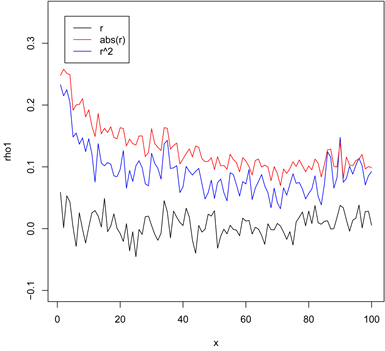(a)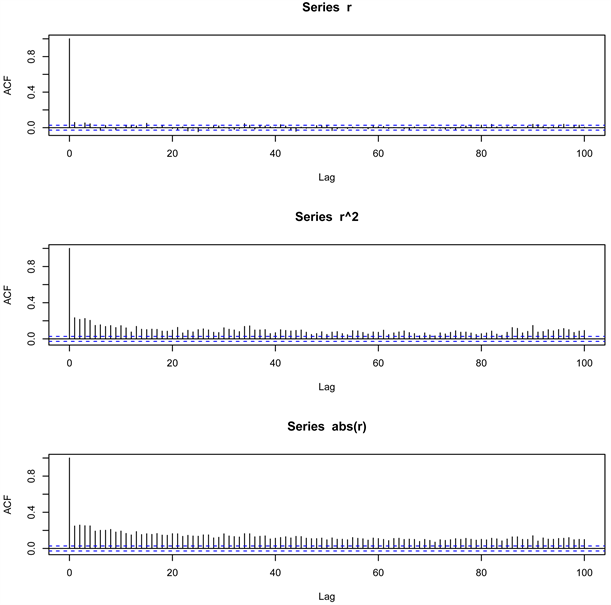(b)

Figure 2. The ACs of the returns, the squared returns and the absolute returns

$\underset{T\to \infty }{\mathrm{lim}}\left(\mathrm{log}{\left(T\right)}^{2}\right)/g\left(T\right)=0$ ，则OLS估计满足以下极限分布：

$\sqrt{m}\left({\stackrel{^}{d}}_{GPH}-d\right)\stackrel{d}{\to }N\left(0,\frac{{\text{π}}^{2}}{24}\right)\text{.}$

3.4. SDS模型的有效性讨论

3.4.1. 模型收益率的自相关结构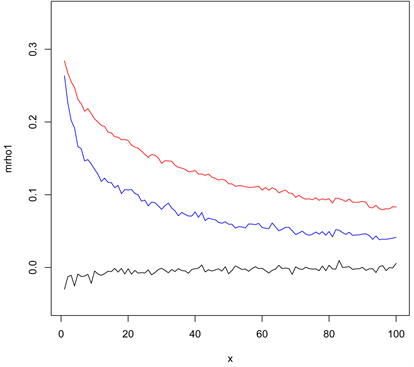Figure 3. The ACs of the returns, the squared returns and the absolute returns for model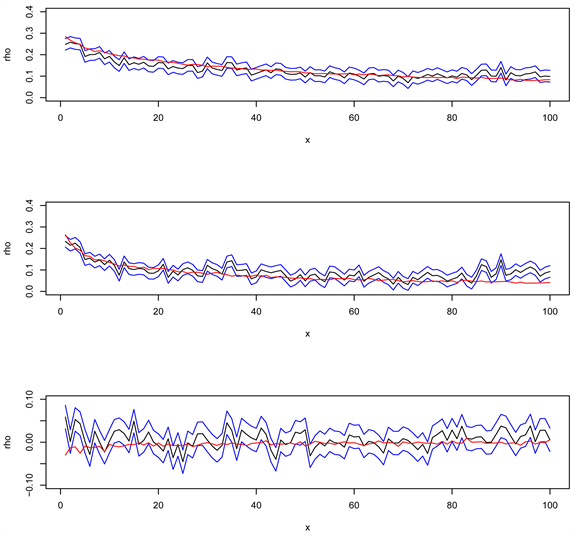Figure 4. The ACs of the returns, the squared returns and the absolute returns for model and the composite

3.4.2. 模型GARCH和TGARCH参数估计

3.4.3. 模型幂率衰减指数估计

4. 结束语

Market Fraction Model with Heterogeneous Agents and Its Empirical Study[J]. 统计学与应用, 2017, 06(04): 460-471. http://dx.doi.org/10.12677/SA.2017.64052

1. 1. Lintner, J. (1969) The Aggregation of Investor’S Diverse Judgments and Perferences in Purely Competitive Security Markets. Journal of Financial & Quantitative Analysis, 4, 347-400. https://doi.org/10.2307/2330056

2. 2. Simon, H. (1957) A Behavioral Model of Rational Choice. In: Models of Man, Social and Rational: Mathematical Essays on Rational Human Behavior in a Social Setting. Wiley, New York. https://doi.org/10.2307/2298010

3. 3. Cutler, D.M., Poterba, J.M. and Summers, L.H. (1991) Speculative Dynamics. Review of Economic Studies, 58, 529- 546.

4. 4. DeLong, J., Shleifer, A., Summers, L. and Waldmann, R. (1990) Noise Trader Risk in Financial Markets. Journal of Economic Analysis & Policy, 98, 703-738.

5. 5. Brock, W. and Hommes, C.H. (1997) A Rational Route to Randomness. Econometrica, 69, 1059-1095. https://doi.org/10.2307/2171879

6. 6. Brock, W. and Hommes, C.H. (1998) Heterogeneous Beliefs and Routes to Chaos in a Sample Asset Pricing Model. Journal of Economic Dynamics and Control, 22, 123-1274. https://doi.org/10.1016/S0165-1889(98)00011-6

7. 7. Bloomfield, R. and Hales, J. (2002) Predicting the Next Step of a Random Walk: Experimental Evidence of Regime-Switching Beliefs. Journal of Financial Economics, 65, 397-414. https://doi.org/10.1016/S0304-405X(02)00147-2

8. 8. Zeeman, E. (1974) On the Unstable Behavior of Stock Exchange. Journal of Mathematical Economics, 1, 39-49. https://doi.org/10.1016/0304-4068(74)90034-2

9. 9. Day, R.H. and Huang, W. (1990) Bulls, Bears and Market Sheep. Journal of Economic Behavior and Organization, 14, 299-329. https://doi.org/10.1016/0167-2681(90)90061-H

10. 10. Kirman, A. (1991) In: Taylor, M. Ed., Epidemics of Opinion and Speculative Bubbles in Financial Markets. Chapter 17 of Money and Financial Markets. Macmillan, London, 354-368.

11. 11. Chiarella, C. (1992) The Dynamics of Speculative Behavior. Annals of Operations Research, 37, 101-123. https://doi.org/10.1007/BF02071051

12. 12. Lux, T. and Marchesi, M. (1999) Scaling and Criticality in a Stochastic Mul-ti-Agent-Model of a Financial Market. Nature, 397, 498-500. https://doi.org/10.1038/17290

13. 13. Chiarella, C., Dieci, R. and He, X. (2009) Heterogeneity, Market Mechanisms and Asset Price Dynamics.

14. 14. He, X. and Li, Y. (2007) Power Law Behavior, He-terogeneity, and Trend Chasing. Journal of Economic Dynamics and Control, 31, 3396-3426. https://doi.org/10.1016/j.jedc.2006.11.008

15. 15. He, X. and Li, Y. (2015) Testing of Market Fraction Model and Power-Law Behavior in the DAX 30. Journal of Economic Finance, 31, 1-17.